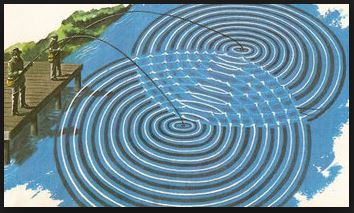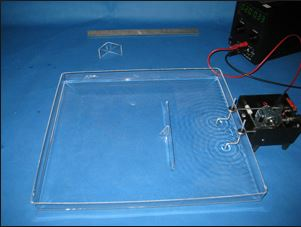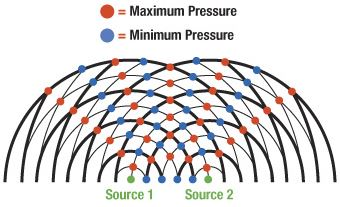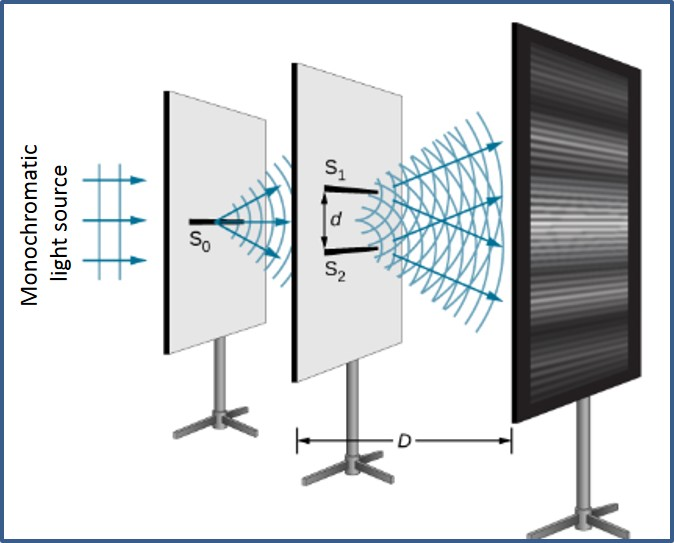# Interference

## Introduction

If two or more waves collide, they are said to interfere. Interference is a property of waves. In this article, how interference occurs in different types of waves is explained.Figure 1: Interference of Waves

A. Interference

If two or more waves overlap, the resultant displacement is the sum of the individual displacements. Displacement is a vector quantity. The overlapping waves are said to interfere. This occurrence is called as interference.

Interference of two waves may lead to a resultant wave of either a larger or a smaller displacement than either of the two component waves. Interference can be demonstrated in a ripple tank by using two-point sources. A ripple tank used to study the interference of two waves is shown in Figure 2.Figure 2: Ripple Tank

Interference of two circular waves is shown in Figure 2. If two waves arrive in phase (their crests arrive at exactly the same time), they will interfere constructively. A resultant wave will be produced, which has crests much higher than the two individual waves, and troughs much deeper.

If the two waves arrive in antiphase (with a phase difference of π radians or 180°), the peaks of one wave arrive at the same time as the troughs from the other, and they will interfere destructively. The resultant wave will have smaller amplitude. Figure 3 shows the interference pattern of two circular waves.

This phase difference may be produced by allowing the two sets of waves to travel different distances – this difference in distance of travel is called the path difference.Figure 3: Interference Pattern of Two Circular Waves

B. Interference of Sound Waves

Interference of sound waves is possible when two or more sound waves having the same frequency collide. The waves with the same frequency (constant phase difference between them) are called coherent waves.

Consider sound waves from two loudspeakers connected to the same signal generator and amplifier, so that each speaker produces a note of the same frequency. Moving about in the space around the speakers, one can hear loud sound where sound waves interfere constructively. In places where the waves interfere destructively, the note is much quieter than elsewhere in the pattern.

C. Interference of Light Waves

Thomas Young demonstrated how light waves could produce an interference pattern using an experiment. A monochromatic light source (a source of one colour, and hence one wavelength λ) is placed behind a single slit to create a small, well-defined source of light. Light from this source is diffracted at the slit (S0), producing two light sources at the double slit (S1 & S2). Because these two light sources originate from the same primary sources, they are coherent and create a sustained and observable interference pattern. The pattern observed on the screen is a series of bright and dark fringes. This experiment is called the Young’s double slit experiment. It is illustrated in Figure 4.Figure 4: Young’s Double Slit Experiment

The distance on the screen between successive bright fringes is called the fringe width. The fringe width is related to the wavelength λ of the light source. It can be calculated by the following equation:Where,

λ – Wavelength of the light source (m).

D – Distance between screen and double slit (m).

d – Distance between centres of two slits S1 & S2 (m).

D. Summary

• The overlapping waves are said to interfere. This occurrence is called as interference.
• If two waves arrive in phase (their crest arrive at exactly the same time), they will interfere constructively.
• If the two waves arrive in antiphase (with a phase difference of π radians or 180°), the peaks of one wave arrive at the same time as the troughs from the other, they will interfere destructively.
• Difference in distance travelled by two waves is called the path difference.

• Waves with the same frequency (constant phase difference between them) are called coherent waves.
• Monochromatic light consists of one colour, and hence one wavelength.
• Young’s double slit experiment is used to study the interference of light waves.
• In Young’s double slit experiment, the distance on the screen between successive bright fringes is called the fringe width. Fringe width can be calculated by the equation (λD)/d.